Next: Earth-Moon-Sun system Up: Lunar motion Previous: Introduction

# Major planetary satellites in solar system

Table 11.1 shows some data for the major planetary satellites in the solar system; that is, the Earth's Moon, the four galilean satellites of Jupiter (Io, Europa, Ganymede, and Callisto), Titan (the principal satellite of Saturn), and Triton (the principal satellite of Nuptune). Although these satellites all have comparable masses, it can be seen that, amongst them, the Moon possesses some fairly unique properties. First, the Moon is much more massive, relative to the planet around which it orbits, than any of the other major satellites. Second, the Moon's orbital radius is significantly larger, relative to the radius of the planet around which it orbits, than those of the other satellites. These facts lead to a number of other unique properties of the Moon. First, on average, the barycenter (i.e., center of mass) of the Earth-Moon system, about which the Earth and the Moon both orbit (see Section 4.16), lies about three-quarters of the radius of the Earth from the Earth's center, leading to a distinct wobble of the Earth, as the Moon revolves around it. Relatively speaking, the satellite-planet barycenters of the other major satellites in the solar system lie much closer to the centers of the planets about which the satellites orbit. Second, the ratio of the mean orbital angular velocity of the Earth around the Sun to that of the Moon around the Earth is much larger than the analogous ratios for the other satellites. As will become clear presently, the square of this ratio is a measure of the lowest-order perturbing action of the Sun on the orbit of the satellite in question. [See Equation (11.38).] Thus, we conclude that this action is much more marked for the case of the Moon than for those of any of the other major satellites in the solar system. Indeed, unlike the Moon, the orbits of the latter satellites are much more strongly affected by planetary oblateness (see Section 10.5) than by solar gravitational perturbations.

Table: Major planetary satellites in the solar system. Here,is the satellite mass,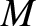the mass of the planet around which the satellite orbits,the major radius of the satellite orbit,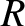the mean radius of the planet,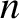the mean orbital angular velocity of the satellite around the planet, and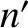the mean orbital angular velocity of the planet around the Sun. The quantity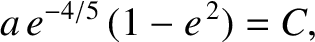denotes the mean radial distance of the satellite-planet barycenter from the center of the planet, relative to the planetary radius. [Source: NASA.]
 Name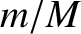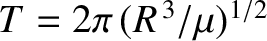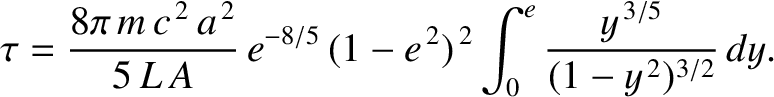Moon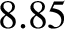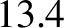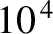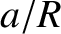Io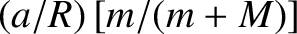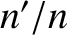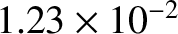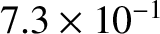Europa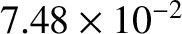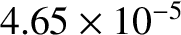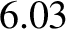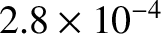Ganymede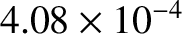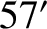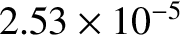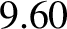Callisto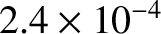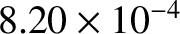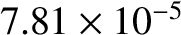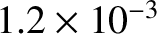Titan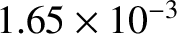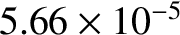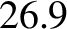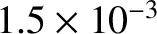Triton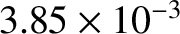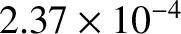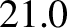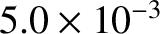Next: Earth-Moon-Sun system Up: Lunar motion Previous: Introduction
Richard Fitzpatrick 2016-03-31